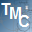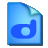# Smart Math Calculator

4.7

CalculatorSmart Math Calculator allows you to define variables and solve math equations. It features a scientific calculator with commonly used functions. This program also lets you define and solve recursive functions. It automatically saves your calculations so you can continue from the last session every time you start the calculator.

4.3
Rated by
6 users
Shareware \$49
OS:
Windows 7/XP/8/8.1/10
Date:
File size:
39.2 MB
Category rank:
16
All software rank:
6 438

## More about Smart Math Calculator

Download Smart Math Calculator v4.7 from SoftDeluxe. This trial version is developed by Runiter Company, Saeid Nourian and has a full version distributed for \$49. It is classified as belonging to the System Utilities category, namely Calculators. This program runs on Windows XP/7/8/8.1 and its most popular versions are 4.2 and 4.1. The statistics show that calculator.exe and calculator_pro.exe are among the most popular setup files for the software.Download
Smart Math Calculator 4.7

## Comments on Smart Math Calculator

Thank you for rating the program!

### Useful softwareMath Calculator
It enables you to calculate expressions and solve equations.